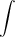Edwin H.

Definite integral from 2 to x vvvv

Find F as a function of x and evaluate it at x = 2, x = 5 and x = 7.2^x (t3 + 6t − 3)dt